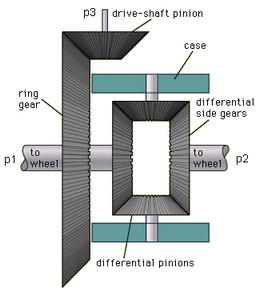﻿ 20-sim webhelp > Library > Iconic Diagrams > Mechanical > Rotation > Gears > DifferentialGear

DifferentialGear

Library

Iconic Diagrams\Mechanical\Rotation\Gears

Use

Domains: Continuous. Size: 1-D. Kind: Iconic Diagrams (Rotation).

Description

A differential gear is well known for its use in automotive mechanics. It transfers power from the engine to the wheels, dividing the force equally between them but permitting them to follow paths of different lengths when turning a corner.When p3 is the drive-shaft and p1 and p3 the shafts connected to the wheels, the constitutive equations for this model are:

p3.omega*2*i = p1.omega + p2.omega;

p1.T = p2.T = p3.T/(2*i);

with i the gear ratio. The gear ratio is equal to the diameter of the drive shaft pinion divided by the diameter of the ring gear, which makes it equivalent to the angular velocity of the drive shaft divided by the average angular velocity of the wheel shafts:

i = p3.omega / ((p1.omega + p2.omega)/2);

Interface

 Ports Description p1 p2,p3 drive shaft port (Rotation) driven shafts ports (Rotation) Parameters i gear ratio []